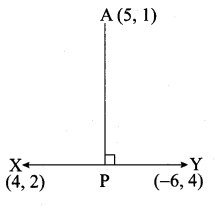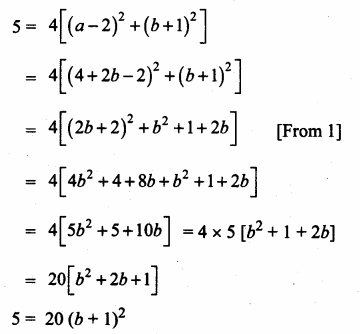## Ex 5.2 | Aster Classes

Question 1.
What is the slope of a line whose inclination with positive direction of x -axis is
(i) 90°
(ii) 0°
Solution:
Here θ = 90°
Slope (m) = tan θ
Slope = tan 90°
= undefined.

(ii) Here θ = 0°
Slope (m) = tan θ
Slope = tan 0°
= 0

Question 2.
What is the inclination of a line whose slope is
(i) 0
(ii) 1
Solution:
(i) m = 0
tan θ = 0 ⇒ θ = 0°
(ii) m = 1 ⇒ tan θ = tan 45° ⇒ 0 = 45°

Question 3.
Find the slope of a line joining the points
(i) (5,5–√) with the origin
(ii) (sin θ, -cos θ) and (-sin θ, cos θ)
Solution:
(i) The given points is (5,5–√) and (0, 0)
Slope of a line = y2−y1x2−x1 = 0−5√0−5
= 5√5=15√

(ii) The given points is (sin θ, -cos θ) and (-sin θ, cos θ)
Slope of a line = y2−y1x2−x1=cosθ+cosθ−sinθ−sinθ
= 2cosθ−2sinθ = – cot θ

Question 4.
What is the slope of a line perpendicular to the line joining A(5,1) and P where P is the mid-point of the segment joining (4,2) and (-6,4).
Solution:
Mid point of XY = (x1+x22,y1+y22) = (4−62,2+42)
= (−22,62) = (-1, 3)Slope of a line = y2−y1x2−x1 = (3−1−1−5)
= 2−6 = – 13

www.asterclasses.com

Question 5.
Show that the given points are collinear: (-3, -4), (7,2) and (12, 5)
Solution:
The vertices are A(-3, -4), B(7, 2) and C(12, 5)
Slope of a line = y2−y1x2−x1
Slope of AB = 2+47+3 = 610 = 35
Slope of BC = 5−212−7 = 35
Slope of AB = Slope of BC = 35
∴ The three points A,B,C are collinear.

Question 6.
If the three points (3, -1), (a, 3) and (1, -3) are collinear, find the value of a.
Solution:
The vertices are A(3, -1), B(a, 3) and C(1, -3)
Slope of a line = y2−y1x2−x1
Slope of AB = 3+1a−3 = 4a−3
Slope of BC = 3+3a−1 = 6a−1
Since the three points are collinear.
Slope of AB = Slope BC
4a−3 = 6a−1
6 (a – 3) = 4 (a – 1)
6a – 18 = 4a – 4
6a – 4a = -4 + 18
2a = 14 ⇒ a = 142 = 7
The value of a = 7

www.asterclasses.com

Question 7.
The line through the points (-2, a) and (9,3) has slope –12 Find the value of a.
Solution:
The given points are (-2, a) and (9, 3)
Slope of a line = y2−y1x2−x1
– 12 = 3−a9+2 ⇒ – 12 = 3−a11
2(3 – a) = -11 ⇒ 6 – 2a = -11
-2a = -11 – 6 ⇒ -2a = -17 ⇒ a = – 172
∴ The value of a = 172

Question 8.
The line through the points (-2, 6) and (4, 8) is perpendicular to the line through the points (8,12) and (x, 24). Find the value of x.
Solution:
Find the slope of the line joining the point (-2, 6) and (4, 8)
Slope of line (m1) = y2−y1x2−x1
= 8−64+2 = 26 = 13
Find the slope of the line joining the points (8, 12) and (x, 24)
Slope of a line (m2) = 24−12x−8 = 12x−8
Since the two lines are perpendicular.
m1 × m2 = -1
13 × 12x−8 = -1 ⇒ 123(x−8)=−1
-1 × 3 (x – 8) = 12
-3x + 24 = 12 ⇒ – 3x = 12 -24
-3x = -12 ⇒ x = 123 = 4
∴ The value of x = 4

www.asterclasses.com

Question 9.
Show that the given points form a right angled triangle and check whether they satisfies Pythagoras theorem.
(i) A(1, -4) , B(2, -3) and C(4, -7)
(ii) L(0, 5), M(9,12) and N(3,14)
Solution:
(i) The vertices are A(1, -4), B(2, -3) and C(4, -7)
Slope of a line = y2−y1x2−x1
Slope of AB = −3+42−1 = 11 = 1
Slope of BC = −7+34−2 = −42 = -2
Slope of AC = −7+44−1 = – 33 = -1
Slope of AB × Slope of AC = 1 × -1 = -1

∴ AB is ⊥r to AC
∠A = 90°
∴ ABC is a right angle triangle
Verification:

20 = 2 + 18
20 = 20 ⇒ Pythagoras theorem verified

(ii) The vertices are L(0, 5), M(9, 12) and N(3, 14)
Slope of a line = y2−y1x2−x1
Slope of LM = 12−59−0 = 79

Slope of MN = 14−123−9 = 2−6 = – 13
Slope of LN = 14−53−0 = 93 = 3
Slope of MN × Slope of LN = – 13 × 3 = -1
∴ MN ⊥ LN
∠N = 90°
∴ LMN is a right angle triangle
Verification:

130 = 90 + 40
130 = 130 ⇒ Pythagoras theorem is verified

Question 10.
Show that the given points form a parallelogram:
A (2.5,3.5), B(10, -4), C(2.5, -2.5) and D(-5, 5).
Solution:
Let A(2.5, 3.5), B(10, -4), C(2.5, -2.5) and D(-5, 5) are the vertices of a parallelogram.

Slope of AB = Slope of CD = -1
∴ AB is Parallel to CD ……(1)

Slope of BC = Slope of AD
∴ BC is parallel to AD
From (1) and (2) we get ABCD is a parallelogram.

www.asterclasses.com

Question 11.
If the points A(2, 2), B(-2, -3), C(1, -3) and D(x, y) form a parallelogram then find the value of x and y.
Solution:
Let A(2, 2), B(-2, -3), C(1, -3) and D(x, y) are the vertices of a parallelogram.

Slope of a line = y2−y1x2−x1
Slope of AB = −3−2−2−2 = −5−4 = 54
Slope of BC = −3+3−2−1 = 0−3 = 0
Slope of CD = y+3x−1
Since ABCD is a parallelogram
Slope of AB = Slope of CD
54 = y+3x−1
5(x – 1) = 4 (y + 3)
5x – 5 = 4y + 12
5x – 4y = 12 + 5
5x – 4y = 17 ……(1)
Slope of BC = Slope of AD
0 = y−2x−2
y – 2 = 0
y = 2
Substitute the value of y = 2 in (1)
5x – 4(2) = 17
5x -8 = 17 ⇒ 5x = 17 + 18
5x = 25 ⇒ x = 255 = 5
The value of x = 5 and y = 2.

Question 12.
Let A(3, -4), B(9, -4) , C(5, -7) and D(7, -7). Show that ABCD is a trapezium.
Solution:
Let A(3, -4), B(9, -4), C(5, -7) and D(7, -7) are the vertices of a quadrilateral.

Slope of a line = y2−y1x2−x1
Slope of AB = −4+49−3 = 06 = 0
Slope of BC = −7+45−9 = −3−4 = 34
Slope of CD = −7+77−5 = 02 = 0
Slope of AD = −7+47−3 = −34 = – 34
The slope of AB and CD are equal.
∴ AB is parallel to CD. Similarly the slope of AD and BC are not equal.
∴ AD and BC are not parallel.
∴ The Quadrilateral ABCD is a trapezium.

Question 13.
A quadrilateral has vertices at A(-4, -2), B(5, -1) , C(6, 5) and D(-7, 6). Show that the mid-points of its sides form a parallelogram.
Solution:
Let A(-4, -2), B(5, -1), C(6, 5) and D(-7, 6) are the vertices of a quadrilateral.

Slope of EF = Slope of GH = 710
∴ EF || GH …….(1)
Slope of FG= Slope of EH = – 712
∴ FG || EH ……(2)
From (1) and (2) we get EFGH is a parallelogram.
The mid point of the sides of the Quadrilateral ABCD is a Parallelogram.

www.asterclasses.com

Question 14.
PQRS is a rhombus. Its diagonals PR and QS intersect at the point M and satisfy QS = 2PR. If the coordinates of S and M are (1, 1) and (2, -1) respectively, find the coordinates of P.
Solution:
Slope of a line = y2−y1x2−x1
Slope of SM = 1+11−2 = 2−1 = -2
Slope of PM = 12 (Since SM and PM are ⊥r)
Let the point p be (a,b)
Slope of PM = 12
b+1a−2 = 12 ⇒ a – 2 = 2b + 2

a – 2b = 4
a = 4 + 2b ……(1)
Given QS = 2PR
QS2 = PR
∴ SM = PR
SM = 2PM (PR = 2PM)

Squaring on both sides∴ (b + 1)2 = 14 ⇒ b + 1 = ± 12
b = 12 – 1 (or) b = – 12 – 1
= – 12 – 1 (or) b = –12 – 1
= – 12 (or) – 32
a = 4 + 2b
a = 4 + 2 (−12)
a = 3
a = 4 + 2 (−32)
a = 4 – 3
a = 1
The point of p is (3,−12) (or) (1,−32)

#### VISITORS COUNTUsers Today : 220Total Users : 166851Views Today : 481Total views : 617294